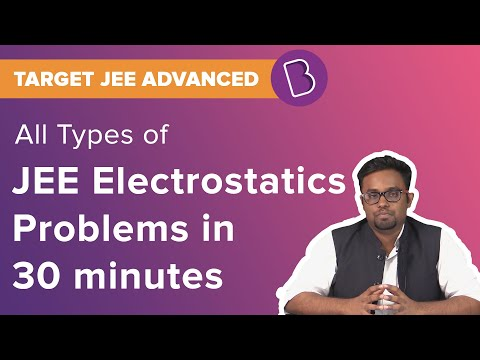# CBSE Class 12 Physics Notes Chapter 2 - Electrostatic Potential and Capacitance

## What Is Electrostatic?

The electrostatic potential is referred to the force which is external but conservative. It is the work done by an external force in bringing a charge s from a point r to a point p. It is the difference in the potential energy of charges between the initial and final points.

Related Topics:

NCERT Solutions for Electrostatic Potential and Capacitance

NCERT Exemplars for Electrostatic Potential and Capacitance

Important Questions for Electrostatic Potential and Capacitance

Capacitor and Capacitance

Electric Potential and Potential Difference

### Potential

The potential of a point is referred to the work done per unit charge in bringing a charge from infinity to a certain point.

### Equipotential Surface

It is a type of surface over which potential has a constant value. When seen as a point charge, concentric spheres centred at a particular location of the charge are equipotential surfaces.

### Potential Energy

The potential energy stored in a system is the work done by the external influence in organizing the charges in their locations.

### Polarisation

The plates of a capacitor enclose a medium which can be filled with insulating substances, and then a net dipole moment is induced by the electric field in the dielectric. This gives rise to the field in the opposite direction.

### Capacitor

A capacitor is a system of two conductors separated by an insulating material.

Students can refer to the short notes and MCQ questions along with separate solution pdf of this chapter for quick revision from the links below:

### Important Question

1. A spherical conductor has a charge of 4 × 10–8C distributed uniformly on its surface of radius 15 cm. Calculate the electric field just outside the sphere and at a point 15 cm from the centre of the sphere.
2. Find out the capacitance if the distance between the plates is reduced by half and the parallel plate capacitor has a capacitance of 20 pF (1pF = 10-12 F) with air between the plates.
3. What is the total capacitance of the combination? Three capacitors, each of capacitance 20 pF, are connected in series.

#### To solve more questions on Electrostatics, watch the below videos## Frequently Asked Questions on Electrostatics

Q1

### An electric dipole has the magnitude of its charge as q, and its dipole moment is p. It is placed in a uniform electric field E. If its dipole moment is along the direction of the field, then what would be the net force acting on it and its potential energy?

If its dipole moment is along the direction of the field, the net force acting on it is zero, and its potential energy is minimum.

Q2

### If E is the electric field intensity of an electrostatic field, then the electrostatic energy density is proportional to?

Electrostatic energy density is proportional to E2.

Q3

### If a charged body is placed near a neutral conductor, then how it will impact the conductor?

In order to maintain the conductor neutral, an exactly equal amount of opposite charge will appear on the opposite surface of the conductor. Thus attractive forces will dominate, and charged body will attract the conductor.